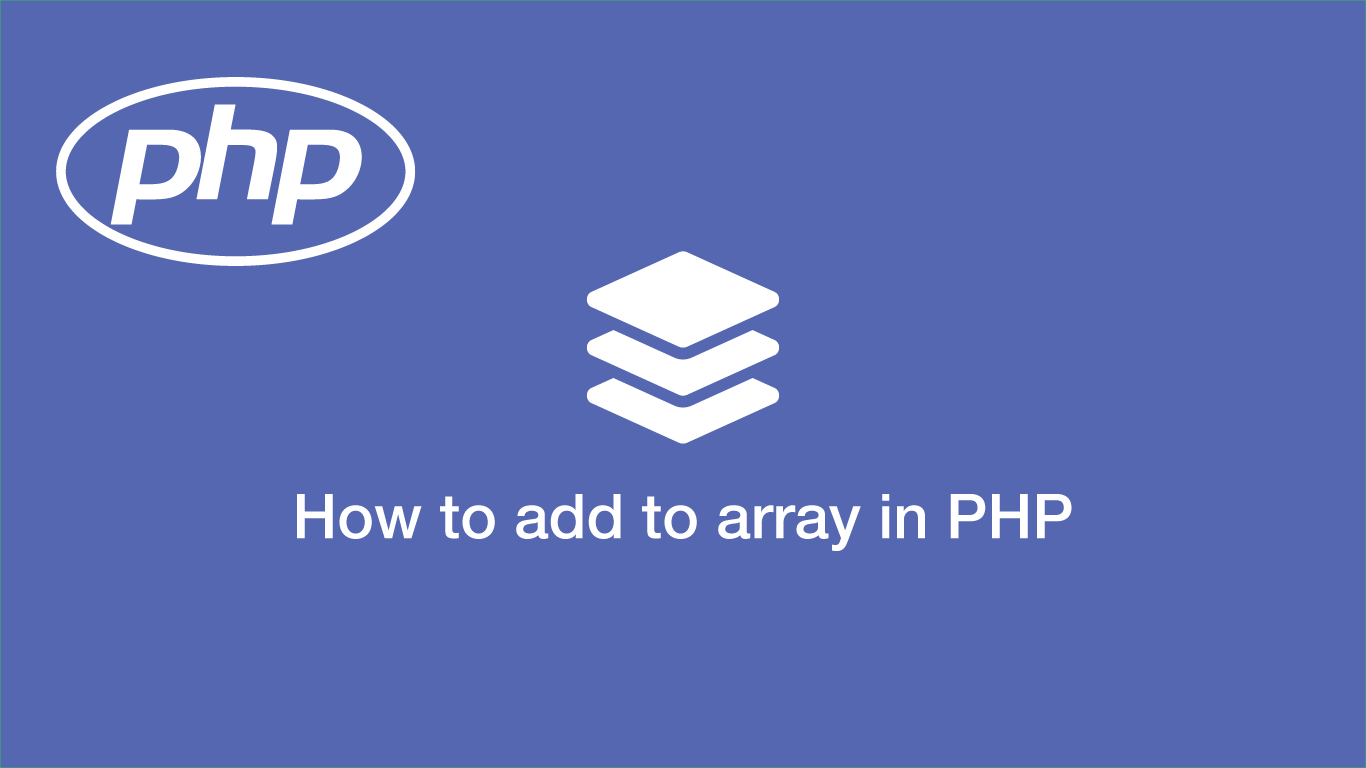# How to Add to an Array in PHPAn array is a collection of data that can be accessed efficiently making them an essential part of working with PHP.

We can work with arrays in several different ways using PHP, let's have a look at how we can declare an array and then explore how we can add and manipulate data in it.

## Creating an Empty Array in PHP

First of all, we want to declare an array as a variable so we can use it. In PHP 5.4 and above an array is declared using the shorthand `[]` (opening and closing square brackets.)

``````\$array = [];
``````

For older versions of PHP, you can use `array()`.

``````\$array = array();
``````

## Pushing a Single Element to an Array

The most basic and efficient way to add data to an array is by pushing a single element.

``````\$array[] = 'foo';
``````

In the above example, we are adding `foo` to key `0` of the array. To add data to a specific key in the array we can specify the number or name of the key within the square brackets.

``````\$array['2'] = 'foo';
``````

## Push a New Element to the End of an Array

Let's as we have the following array:

``````\$array = ['a','b','c'];
``````

If we want to add `d` to the end of the array, we can use the PHP `array_push()` utility. This takes two arguments, firstly the array to use and then the data to add.

``````array_push(\$array, 'd');
``````
``````['a','b','c','d']
``````

## Push a New Element to the Start of an Array

To push an element to the beginning of an array, thus shifting all the existing elements up one key we can use the PHP `array_unshift()` utility. It takes two arguments, the array and then the data to be pushed.

``````array_unshift(\$array, 'd');
``````
``````['d','a','b','c']
``````

## Push a New Element to a Specified Key in the Array

If we need to add a new element in a specific position we can use the PHP `array_splice()` utility. The first argument is the existing array, secondly the key where the item(s) are to be added from, thirdly the number of keys to remove and lastly the array of elements to add.

``````\$array = ['a','b','c'];
``````

Let's say we want to add `d` and `e` before `c` in the above array. The key of `c` is `2` since the array starts at key `0`. To get the desired result we could use the following `array_splice()` code:

``````array_splice(\$array, 2, 0, ['d', 'e']);
``````

## Adding Multiple Elements to an Array

Adding multiple elements to an array can be done using a `for` loop.

``````\$array = [];

for (\$i=0; \$i < 5; \$i++) {
\$array[\$i] = \$i;
}
``````

In the above example, we are looping 5 times and matching the key of our `for` loop with an array key (`[\$i]`) and then setting the data as the for loop key also.

``````['0','1','2','3','4']
``````

You can also use `foreach` or `while` to cycle through existing data and build a new array from them. Let's assume we have a PHP collection of dog information containing the property of name, we could build a new array of dog names like this:

``````\$array = [];

foreach (\$dogs as \$key => \$d) {
\$array[\$key] = \$d->name;
}
``````

## Building a Multidimensional Array

We can build a multidimensional array by nesting `for` loops. Let's say we wanted to build a multidimensional array with a set number of items and nested elements.

``````\$array = [];
\$items = 5;
\$nested = 5;

for (\$i=0; \$i < \$items; \$i++) {
for (\$w=0; \$w < \$nested; \$w++) {
\$array[\$i][\$w] = \$w;
}
}
``````

If we wanted to add data to elements we just have to specify the top-level array key followed by the nest one.

``````\$array['2']['3'] = 'foo';
``````

## Conclusion

You now know a variety of ways to add elements to arrays in PHP.

#### Related Tutorials### How to Remove Elements from Arrays in PHP

December 17, 2020### How to Use Associative Arrays in PHP

September 06, 2020### How to Create and Use Lists (Arrays) in Python

September 01, 2020### How to Encode, Decode and Use JSON Data in PHP

September 06, 2020### How to Count all Elements in an Array in PHP

December 21, 2020March 23, 2021